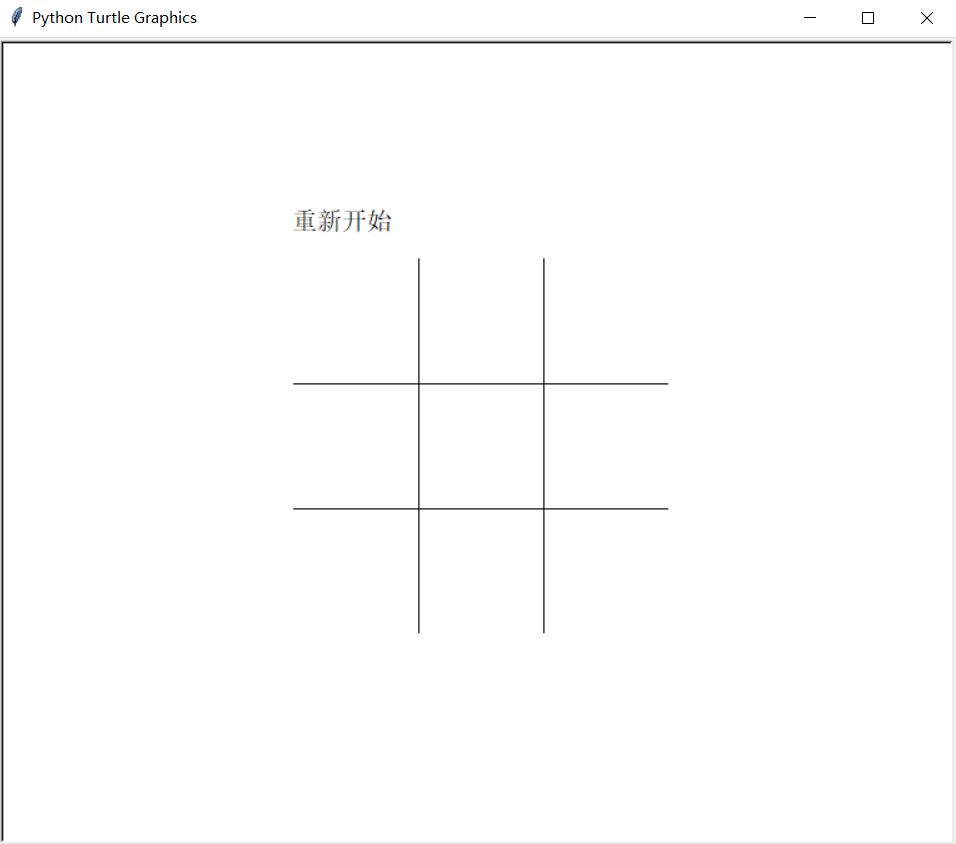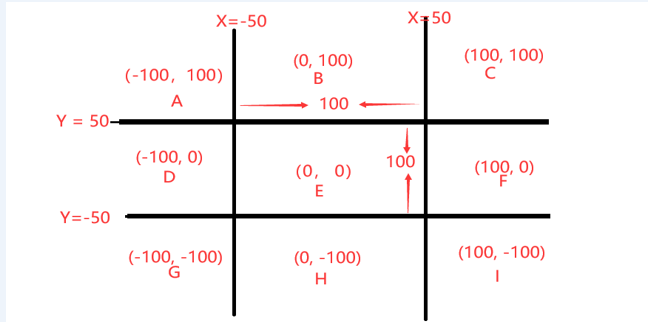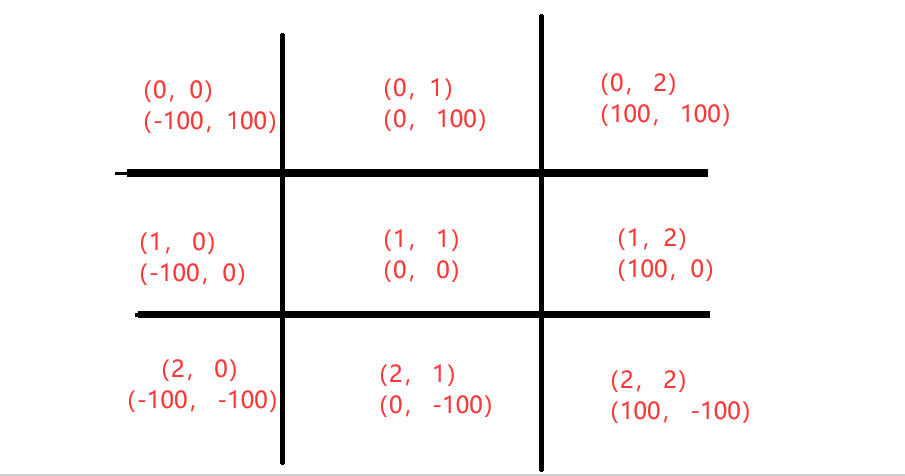[[d.project_title]][[d.material_name]]# mind+python井子棋游戏

1设计背景、功能需求

学生已经掌握了海龟模块的基本使用，以及一些常用的函数，能否使用一款游戏让学生对该模块进行实践。结合海龟模块的屏幕时间，可以设计一个双人对战的井字棋游戏。该游戏需要具备以下功能：棋盘的初始化，通过鼠标点击的位置在棋盘对应的区域画一颗棋子，根据点击次数模拟两人对战的情况，判断输赢结果，重新开始等功能。

2功能原理

本项目的界面使用的是Turtle模块实现的，使用Turtle模块的中的up、goto、down、fd等命令实现棋盘的初始化。Turtle模块的Screen对象绑定鼠标点击后的回调函数实现鼠标点击后需要完成的动作。通过x、y坐标将棋盘分为九个区域，只有鼠标点击的位置在棋盘区域棋盘上则会判断该区域是否存在棋子不存在则在该区域的中心画一颗棋子，反之不执行任何操作。将棋盘的映射到一个二维列表中，当棋盘每下一颗棋子就将该区域在二维列表对应下表的值改变。通过遍历该二维列表判断是否有存在三颗一样的棋子，存在则在棋盘上输出结果游戏结束。不存在三颗一样的棋子判断是否棋盘的每个区域都有棋子，如果都有棋子则在棋盘上显示伯仲之间，反之继续游戏。在游戏的图中可以通过重新开始，开始新一轮。

3详细步骤

3.1界面介绍：界面有两个部分组成棋盘和重新开始的按钮如图3.1.1所示。

3.2 棋盘每个区域中心位置坐标分析，图3.1.1中的棋盘没根线的之间的距离为100，横线做最左边的x为-100，竖线最上边y为150，每个区域中心坐标如图3.2.1所示。根据鼠标点击棋盘的区域的坐标将鼠标点击的坐标转换成每个区域的中心坐标，为后面的棋盘到二维列表的映射做铺垫以及保证下的每颗棋子在每个区域的中心。

3.3棋盘区域中心坐标映射到二维列表中，如图3.3.1所示

3.4胜负判断方法：将井字棋的每个区域的坐标映射到二维列表中去，通过循环访问该列表的方法判断是否有人获胜。通过观察二维列表索引的变化情况可以发现具能取胜的情况有四种分别为横，竖，左斜，右斜四种情况，每种情况又分为棋手下的棋的正半段和负半段，正负段判断结束为当取的格子的棋子颜色和判断棋子的颜色不同则退出，相同就将该坐标添加到表中，正负半段判断完后，退出第二层循环```	                    					```import turtle as t
import time
def draw():
'''
画棋盘
'''
# 重新开始
t.up()
t.goto(-150, 170)
t.write('重新开始', font=('', 15))
for i in range(2):
t.up()
t.goto(-150, 50-i*100)
t.down()
t.fd(300)
t.seth(-90)
for i in range(2):
t.up()
t.goto(-50+i*100, 150)
t.down()
t.fd(300)
t.up()
def victory(x, y, num):
'''
x：int表示现在下的棋子的横坐标
y：int表示现在下的棋子的纵坐标
num：int表示下棋者，1表示红棋2表示蓝棋
'''
global zb_, stop
# 判断是否取得胜利
# 将图中的位置映射到zb_这个列表中, r表示行，c表示列
if y == 100:
r = 0
elif y == 0:
r = 1
else:
r = 2
if x == -100:
c = 0
elif x == 0:
c = 1
else:
c = 2
# 将棋子映射到列表中
zb_[r][c] = num
print(zb_)
# 判断周围是否赢棋 只有棋子的总数大于等于五颗才判断是否有人获得胜利
if len(zb) < 4:
return False
# 判断棋的周围是否有三颗相同的棋
for i in range(4): # 先判断行，在列然后斜着
# 用于判断是否有三个一样的
num_qz = [(r, c)]
for j in range(2): # 根据坐标分为两种情况
r_ = r
c_ = c
# 第一次判断左边有几颗相同的棋
while zb_[r_][c_] == num:
if i == 0 and j == 0:
r_ -=  1
elif i == 0 and j == 1:
r_ += 1
elif i == 1 and j == 0:
c_ -= 1
elif i == 1 and j == 1:
c_ += 1
elif i == 2 and j == 0:
r_ -= 1
c_ -= 1
elif i == 2 and j == 1:
r_ += 1
c_ += 1
elif i == 3 and j == 0:
r_ += 1
c_ -= 1
else:
r_ -= 1
c_ += 1
if r_ > 2 or r_ < 0 or c_ > 2 or c_ < 0:
# 遍历完了整个棋盘
break
if zb_[r_][c_] == num:
num_qz.append((r_, c_))
if len(num_qz) == 3:
stop = True
return True, num, num_qz
return False

def panduan(x, y):
x_ = x
y_ = y
# 判断x和y在哪个范围
if -150 <= y < -50:
y_ = -100
elif -50 <= y < 50:
y_ = 0
elif 50<= y < 150:
y_ = 100

if -150< x <= -50:
x_ = -100
elif -50< x <= 50:
x_ = 0
elif 50 < x <= 150:
x_ = 100
if x_ == x or y_ == y:
return False
else:
return x_, y_

def func(x, y):
'''
鼠标点击事件
'''
print(x, y)
global num, zb, stop
print(15)
if (-174 < x < -72 and 174 < y < 188):
print(454)
t.home()  # 要保证笔头朝向正东
stop = False
t.clear()
start()
return
if not stop:
if len(zb) == 9:
return
res = panduan(x, y)
if res:
x = res
y = res
if (x, y) in zb:
return
t.goto(x, y)
if num % 2 == 0:
print(111)
t.dot(80, 'red')
# 判断是否胜利
res = victory(x, y, 1)
else:
t.dot(80, 'blue')
res = victory(x, y, 2)
zb.append((x, y))
# 判断是否胜利
if res:
# t.clear()
t.goto(-125, -25)
if res == 1:
# t.color('red')
t.write('红棋获得胜利', font=('楷体', 30))
else:
# t.color('blue')
t.write('蓝棋获得胜利', font=('楷体', 30))
elif len(zb) == 9:
# t.clear()
t.goto(-90, -25)
t.write('伯仲之间', font=('楷体', 30))
stop = True
num += 1

def start():
global stop, zb, zb_, num
stop = False
# 定义一个点击次数用于判断点击了几次，用于下不同的棋子
num = 0
# 定义一个列表存放下过的坐标点
zb = []
# 定义一个二位列表用于判断是否取得胜利1表示红色2表示蓝色
zb_ = [[0, 0, 0], [0, 0, 0], [0, 0, 0]]

# 定义屏幕对象
draw()

t.tracer(False)
t.ht()
start()
screen = t.Screen()
screen.onscreenclick(func)
t.mainloop()
t.tracer(True)

```
```

```	                    					`<iframe frameborder="0" src="https://v.qq.com/txp/iframe/player.html?vid=z32765r9r96" allowFullScreen="true"></iframe>`
```
Makelog作者原创文章，未经授权禁止转载。
3
1[[c.user_name]] [[c.create_time]]
[[c.parent_comment.count]]
|
[[c.comment_content]]
[[r.user.user_name]] [[r.create_time]]
[[r.comment_content]]

3
11
0
10
1
4
1
5
2
5